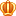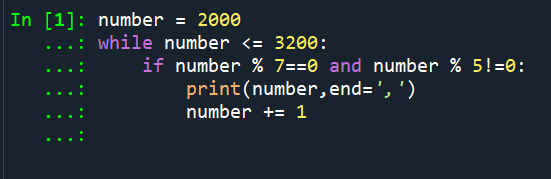##帐号 自动登录 找回密码 密码 立即注册
 搜索

# [已解决]求助while语句无法运行也不报错发表于 2020-10-15 05:39:23 | 显示全部楼层 |阅读模式

### 马上注册，结交更多好友，享用更多功能^_^

xnumber = 2000
while number <= 3200:
if number % 7==0 and number % 5!=0:
print(number,end=',')
number += 1

想知道哪里错了，在结尾加了break 或者 continue都不行~2020-10-15 07:14:12
1. number = 2000
2. while number <= 3200:
3.     if number % 7==0 and number % 5!=0:
4.         print(number,end=',')
5.     number += 1 # 这里要减少一级缩进，与if同级，表示不论是否符合if条件，都会执行number+=1，你原来的写法是只有符合if条件才会加1，导致一开始第一个不符合条件的数就不能加1而一直死循环。发表于 2020-10-15 07:09:07 From FishC Mobile | 显示全部楼层
 number+=1放在if语句里是神马鬼发表于 2020-10-15 07:14:12 From FishC Mobile | 显示全部楼层    本楼为最佳答案
 .pct{min-height:100px;}number = 2000 while number <= 3200:     if number % 7==0 and number % 5!=0:         print(number,end=',')     number += 1 # 这里要减少一级缩进，与if同级，表示不论是否符合if条件，都会执行number+=1，你原来的写法是只有符合if条件才会加1，导致一开始第一个不符合条件的数就不能加1而一直死循环。复制代码发表于 2020-10-15 09:00:42 | 显示全部楼层
 number = 2000                      while number <= 3200:     if number % 7==0 and number % 5!=0:              print(number,end=',')         number += 1                         #if的结果为True，则会运行这行，否则不运行。你应该把number的缩进和if对齐发表于 2020-10-15 13:57:44 | 显示全部楼层
 这就是我们初学者常犯的错误，对齐的位置还是非常重要的，继续加油发表于 2020-10-15 14:46:00 | 显示全部楼层
 number = 2000                      while number <= 3200:     if number % 7==0 and number % 5!=0:              print(number,end=',')         number += 1                     这个小程序只会执行一次number等于2000,就会退出了，因为2000不符合if为True 的输出，而number+1在这个程序中亦属于if 条件为True时才能执行，所以什么输出都没有；number+1要 在 if 程序段外且在while程序段里执行才能生效。发表于 6 天前 | 显示全部楼层
 缩进闹得鬼

 您需要登录后才可以回帖 登录 | 立即注册 本版积分规则 回帖后跳转到最后一页

GMT+8, 2020-10-23 05:15